# Force and Pressure Class 8 Notes - Chapter 11

According to the CBSE Syllabus 2023-24, this chapter has been renumbered as Chapter 8.

## Introduction

In science, a push or pull of an object is known as force. The interaction between two objects arises force. Force has both magnitude and direction. The strength of a force is expressed in magnitude. Force brings about a change in the direction or state of motion of a body. In Class 8 Science Chapter 11, various characteristics of force, along with the types of force are discussed.

### Push

A push is a force exerted away from the body, for example, Hitting a snooker ball, or kicking a football.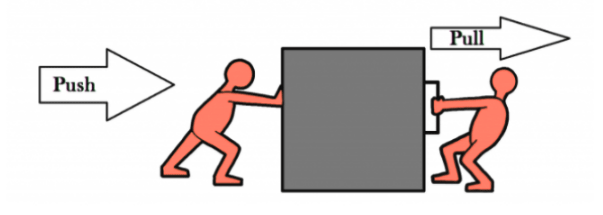#### For more information on Force – A Push or A Pull, watch the below video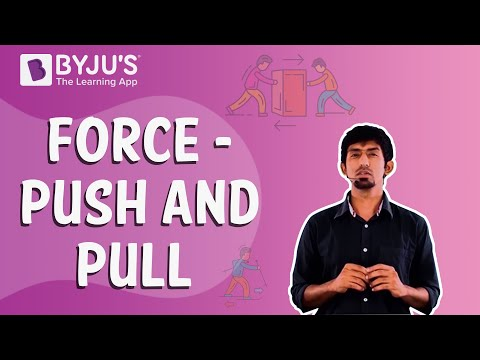To know more about “Force – Pull and Push”, visit here.

### Magnetic force

The force of attraction or repulsion between two magnetic bodies due to their poles is known as a magnetic force.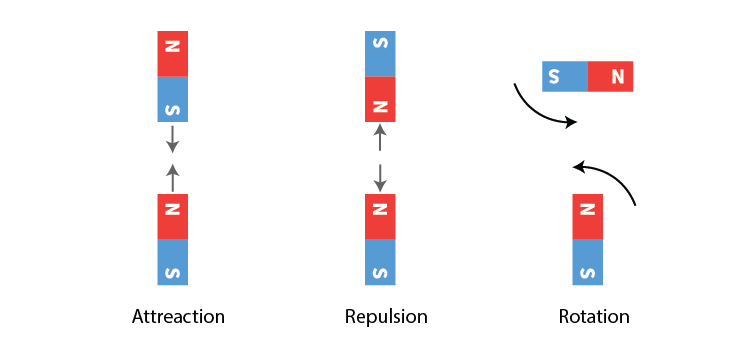To know more about Magnetic Force, visit here.

## What Is It?

### Pull

A pull is a force exerted towards the body, for example, drawing a bucket of water from a well or playing tug of war.

### Force

• A force is a push or a pull.
• The interaction between objects can change the state of the objects.

To know more about Force, visit here.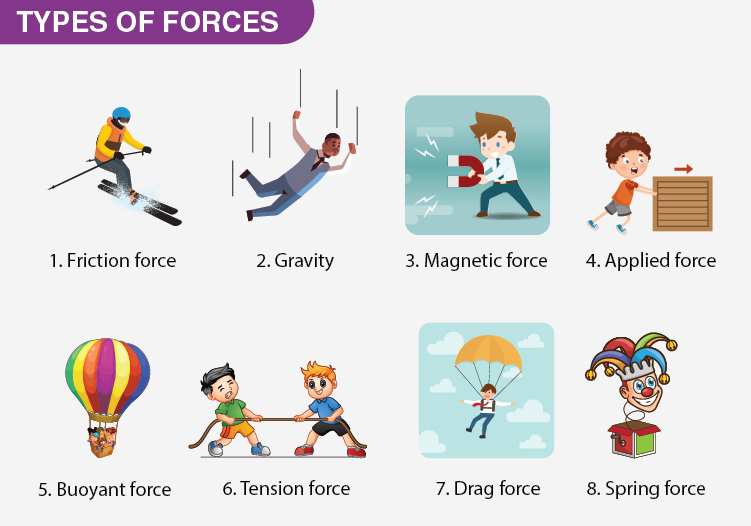#### For more information on Introduction to Force and Its Types, watch the below video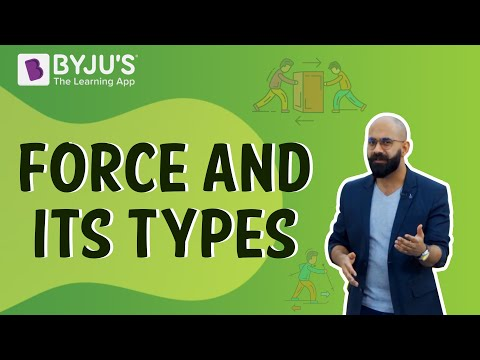To know more about Types of Force, visit here.

## Playing With It

### Net force

• The result of all the forces acting on a body is known as the net force.
• The acceleration of the body is along the direction of the net force.

### Frictional force

• The force that opposes the relative motion between two surfaces.
• Acts between the surface of the two bodies in contact.
• Type of contact force.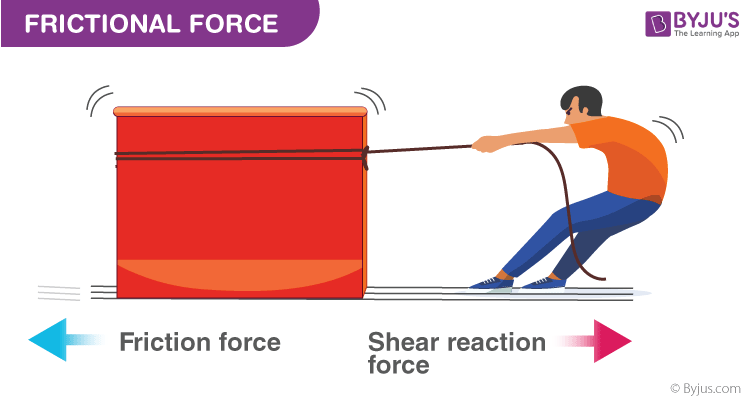To know more about Frictional Force, visit here.

## What Can Force Do?

### Vector

– Vector quantities are expressed in magnitude as well as the direction of the object. For example, velocity, displacement, weight, momentum, force, acceleration etc.
– Vectors are used to find the resultant component acting on a body.
– When multiple forces act on a body, they can be resolved into one component known as the net force acting on the object.
Example: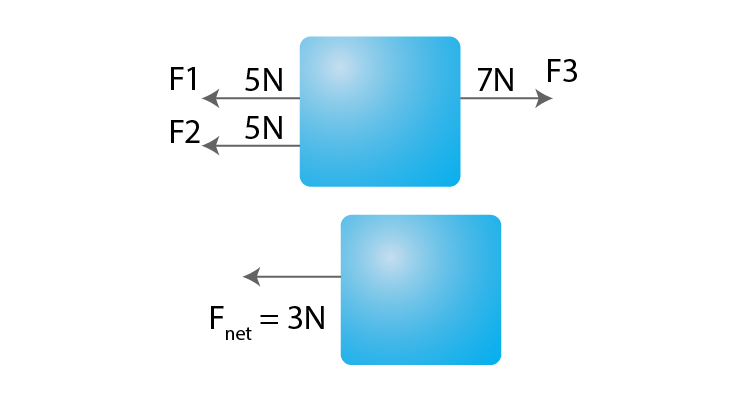Vectors are also useful when the force acts at an angle to the horizontal.

### Application of Force

– A force is an effort that changes the state of an object at rest or in motion.
– It can change an object’s direction and velocity.
– Force can also change the shape of an object.

### State of Motion

The state of motion of an object is defined by its velocity – the speed with a direction. Thus, inertia could be redefined as follows:
Inertia = tendency of an object to resist changes in its velocity.
An object at rest has zero velocity – and (in the absence of an unbalanced force) will remain with a zero velocity; it will not change its state of motion (i.e., velocity). An object in motion with a velocity of 2 m/s, East; will (in the absence of an unbalanced force) remain in motion with a velocity of 2 m/s, East; it will not change its state of motion (i.e., velocity). Objects resist changes in their velocity.

## Types of Forces

### Contact

Touch or contact is required to do most of our everyday activities. For example, lifting, pulling, kicking, pushing etc.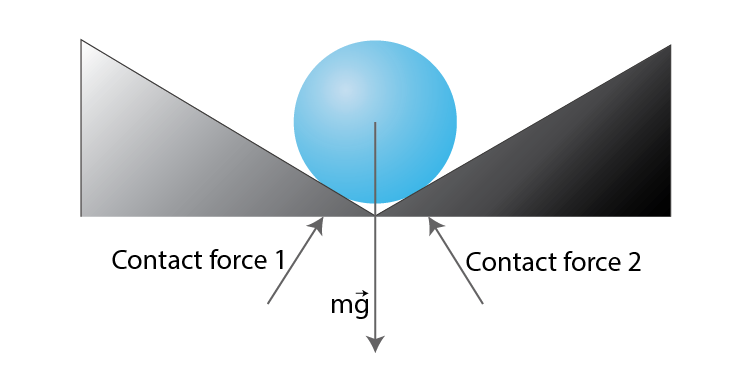### Contact forces

Forces that require a touch or contact to be applied are known as contact forces. For example, muscular forces and frictional forces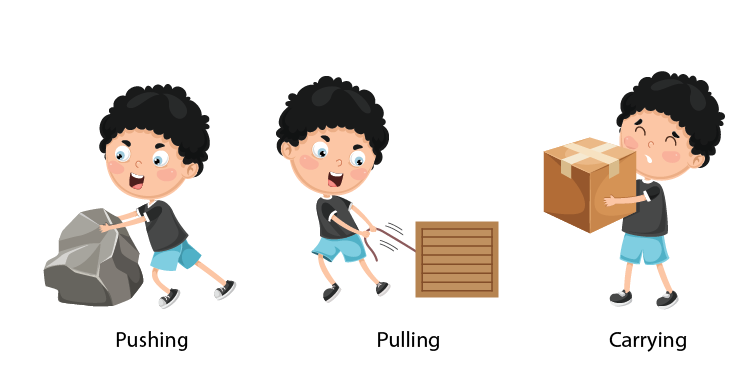### Muscular force

The force applied by the effort of our muscles, for example, lifting a heavy box, pulling a bucket of water or pedalling a cycle.

### Non-contact forces

• Forces that do not need contact or that have their influence without a touch. For example, magnetic force, electrostatic force, and gravitational force.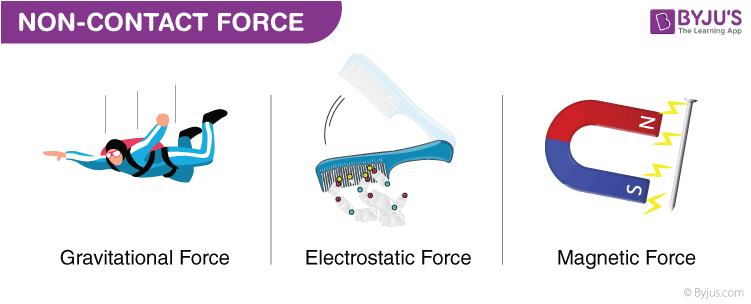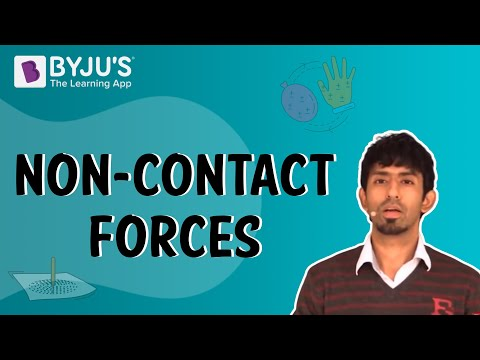### Gravitational force

• The attractive force that a body experiences towards the centre of the earth is called the force of gravity due to earth.
• Property of the universe, every object attracts or exerts a force on every other object.

### Electrostatic force

The force of attraction or repulsion experienced by a charged body from another charged body in the same vicinity is known as Electrostatic Force.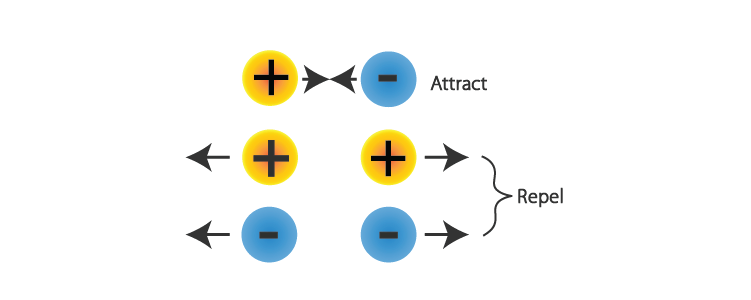### Nuclear forces

• The nuclear force acts between all the particles in the nucleus. i.e., between two neutrons, between two protons and between a neutron and a proton.
• It is an attractive force in all cases.
• It is the force that keeps the nucleus intact by overcoming the enormous repulsive force between positive protons.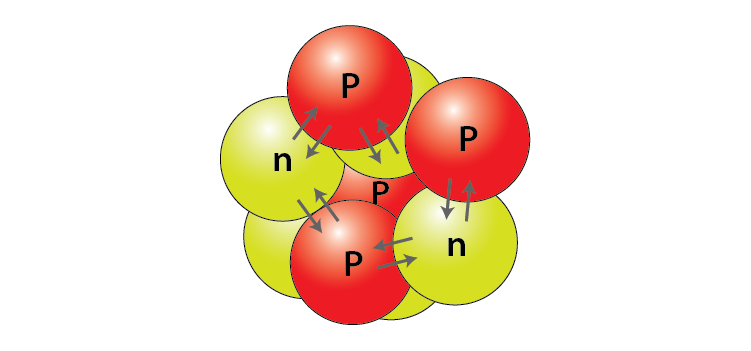## Thrust and Pressure

### Pressure

• Force acting per unit area is known as pressure.
• $$\begin{array}{l}P=\frac{force}{area}\end{array}$$
• SI unit is Pascal.

#### For More Information On Thrust and Pressure, Watch The Below Video: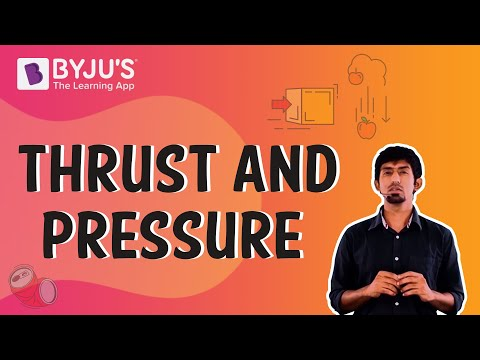To know more about Thrust and Pressure, visit here.

### Distribution of pressure

• Force acting on a smaller area applies more pressure than the same force acting on a larger area.
• Examples: Porters place a round cloth on their heads to increase surface area and reduce pressure.
• A sharp knife cuts better as more pressure is exerted over a smaller area.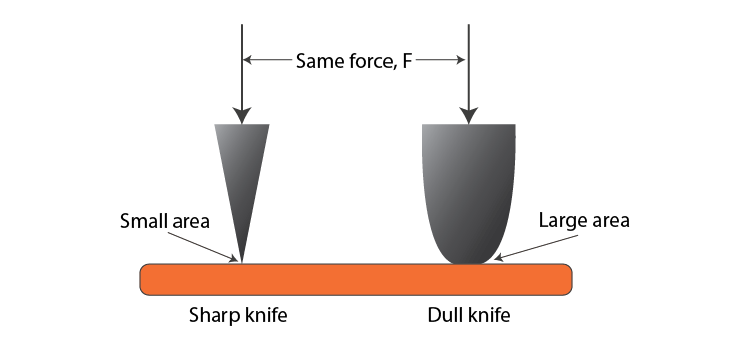### Pressure in fluids

The pressure exerted by a fluid(gases or liquids) in a container is transmitted undiminished in all direction on the walls of the container.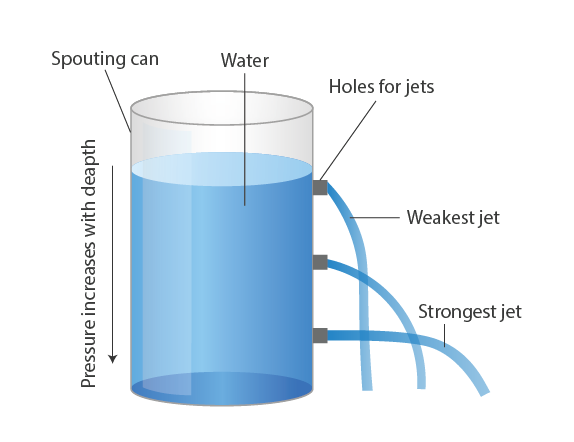To know more about Fluid Pressure, visit here.

### Upthrust

The upward force exerted by a fluid on an object is known as upthrust or buoyant force.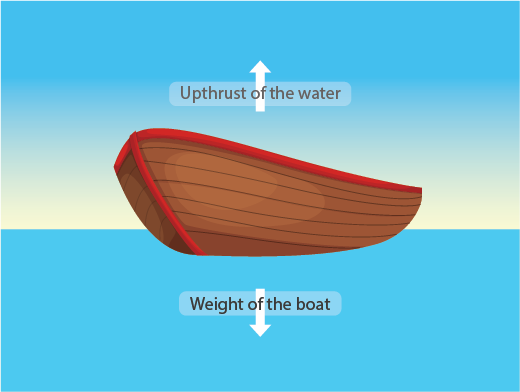## Atmospheric Pressure

### Gaseous pressure

Gases exert the same pressure on the walls of the container in all directions.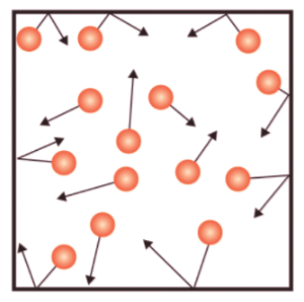### Atmospheric pressure

• Our atmosphere extends to several kilometres above sea level. The weight of the air acts as pressure known as atmospheric pressure.
• The pressure in our body balances the atmospheric pressure and that is why we do not feel it.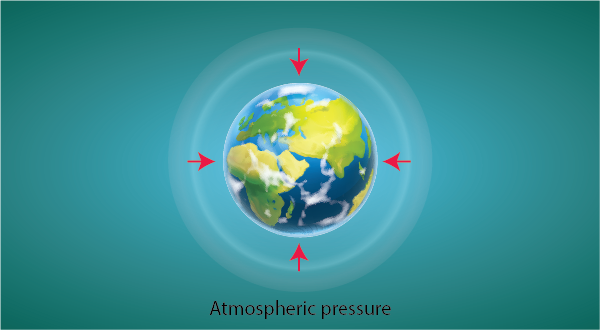To know more about Atmospheric Pressure, visit here.

 Also Access NCERT Solutions for Class 8 Science Chapter 11 NCERT Exemplar for Class 8 Science Chapter 11

Learn more about force and its various characteristics and other related topics, including class 8 Science notes, at BYJU’S.

 Types of Force Force and Motion

## Frequently Asked Questions on CBSE Class 8 Science Notes Chapter 11 Force and Pressure

Q1

### What is ‘Spring force’?

Spring force is a restoring force that tends to retract the spring back to its original position.

Q2

### What are Non-contact forces?

A non-contact force is a force which acts on an object without coming physically in contact with it.

Q3

### What is ‘Thrust’?

Thrust is defined as quickly pushing with force.